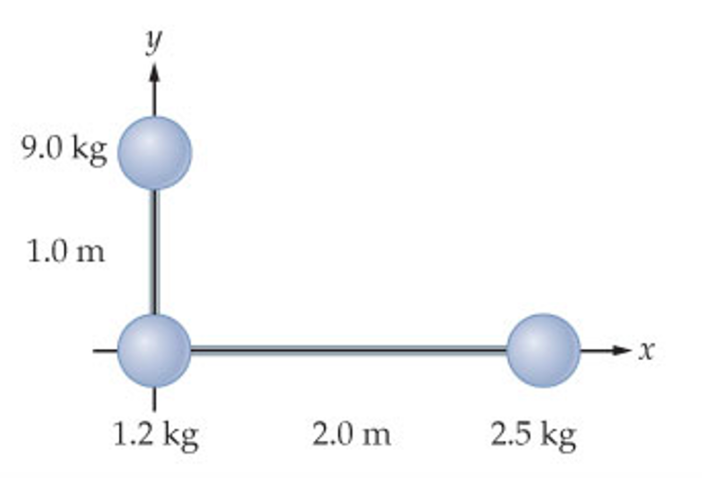# Problem: The L-shaped object in the figure (Figure 1) consists of three masses connected by light rods.What torque must be applied to this object to give it an angular acceleration of 1.00 rad/s2 if it is rotated about the y-axis?

###### FREE Expert Solution

Moment of inertia of a point mass:

$\overline{){\mathbf{I}}{\mathbf{=}}{\mathbf{m}}{{\mathbf{r}}}^{{\mathbf{2}}}}$

Torque and angular acceleration:

$\overline{){\mathbf{\tau }}{\mathbf{=}}{\mathbf{I}}{\mathbf{·}}{\mathbf{\alpha }}}$

We have only one mass that lies outside the y-axis.

93% (376 ratings)###### Problem Details

The L-shaped object in the figure (Figure 1) consists of three masses connected by light rods.What torque must be applied to this object to give it an angular acceleration of 1.00 rad/s2 if it is rotated about the y-axis?